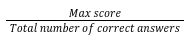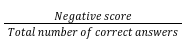Help Center
1. Help Center
2. Assessments: Recruiters
3. Multiple Choice Questions (MCQs)

# Partial scoring

HackerEarth has introduced partial scoring in the MCQ questions with multiple correct answers. Partial scoring is a concept of providing scoring on the basis of the number of correct answers. It will also equally distribute the Maximum and Negative scores across correct and incorrect options, respectively. Partial scoring is only available if you allow multiple answers in MCQ. You can easily enable partial scoring in MCQs with multiple answers by selecting the check box stating ‘Allow partial scoring’.

Scoring for Partial scoring

The scoring is as follows:

• Candidates receivefor each correct option selected.
• Candidates receivefor each incorrect option selected.
• Candidates receive a negative score or 0 (if negative scoring is not enabled) for the entire question if candidates select more than the total number of correct options.
Example of partial scoring

For example, an MCQ with partial scoring has the following settings:

• Max score: 4
• Negative score: 2
• Available choices:  A, B, C, and D
• Correct choice: A and C
 Candidate’s answer Assigned score Explanation A (One correct) 2 The correct option earns 4/2 = 2 points for a total of 2 points B (One incorrect) -1 The incorrect option earns -2/2 = -1 point for a total of -1 point A & C (Both correct) 4 Each correct option earns 4/2 = 2 points for a total of 2x2 = 4 points A & B (One correct and one incorrect) 1 The correct option earns 4/2 = 2 points, and the incorrect one earns -2/2 = -1 points for a total of 2 - 1 = 1 point B & D (Both incorrect) -2 Each incorrect option earns -2/2 = -1 points for a total of 2 x -1 = -2 points A, C & D -2 Since the candidate has selected more than the number of correct options available (2) the candidate will receive the full negative score of -2